# NCERT Solutions for Class 12 Chemistry Chapter 9 Coordination Compounds

Here we provide NCERT Solutions for Class 12 Chemistry Chapter 9 Coordination Compounds for English medium students, Which will very helpful for every student in their exams. Students can download the latest NCERT Solutions for Class 12 Chemistry Chapter 9 Coordination Compounds pdf, free NCERT solutions for Class 12 Chemistry Chapter 9 Coordination Compounds book pdf download. Now you will get step by step solution to each question.

## NCERT Solutions for Class 12 Chemistry Chapter 9 Coordination Compounds

Complex compounds are known as coordination compounds. It is an important chapter from the examination perspective. The students need to be thorough with the concepts such as properties, examples and applications of coordination compounds.

A thorough knowledge of the basic concepts helps the students while studying the advanced concepts. This not only helps them during boards and home exams but also during competitive exams.

NCERT IN-TEXT QUESTIONS

Question 1.
Write the formulas of the following co-ordination compounds :
(a) tetraamminediaquacobalt (III) chloride
(b) potassium tetracyanonickelate (II)
(c) tris (ethane-1, 2-diamine) chromium (III) chloride
(d) amminebromidochloridonitrito-N-platinate (II)
(e) dichlorobis (ethane-1, 2-diamine) platinum (TV) nitrate
(f) iron (III) hexacyanoferrate (II).
(a) [Co(NH3)4(H2O)2]Cl3
(b) K2[Ni(CN)4]
(c) [Cr(en)3]Cl3
(d) [Pt(NH3)BrCl(NO2)]
(e) [PtCl2(en)2](NO3)2
(f) Fe4[Fe(CN)6]3.

Question 2.
Write the IUPAC names of the following coordination compounds:
(i)[Co(NH3)6]Cl3
(ii)[Co(NH3)5Cl]Cl2
(iii)K3[Fe(CN)6l
(iv)K3lFe(C2O4)3]
(v)K2[PdCl4]
(vi)[Pt(NH3)2Cl(NH2CH3)]Cl
(i) Hexaamminecobalt (III) chloride
(ii)Pentaamminechloridecobalt (III) chloride
(iii)Potassium hexacyanoferrate (III)
(iv)P9tassiumtrioxalatoferrate(III)
(vi)Diamminechloride (methylamine) platinum (II) chloride

Question 3.
Give the types of isomerism exhibited by the following complexes and draw the structures of these isomers :
(a) K[Cr(H2O)2(C2O4)2]
(b) [Co(en)3]Cl3
(c) [CO(NH3)5(NO2)] (NO3)2
(d) [Pt(NH3) (H2O)Cl2] (C.B.S.E. Outside Delhi 2013)
(a) K[Cr(H2O)2(C2O4)2] or K[Cr(H2O)2(OX)2]
(i) It exists as geometrical isomers :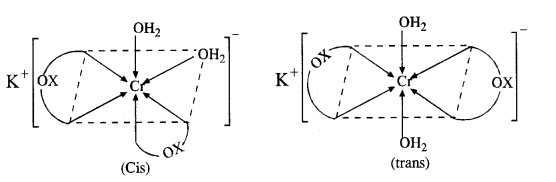(ii) The cis isomer can also exist as pair of optical isomers.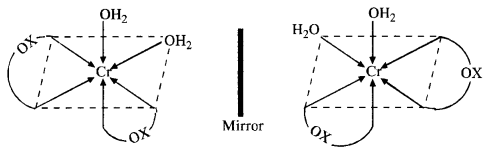(b) The complex can exist as optical isomers. For structure, consult section 9.7.
(c) The complex can exist as pair of ionisation isomers as well as linkage isomers. Ionisation isomers : [Co(NH3)5(NO2)] (NO3)2 and [Co(NH3)5(NO3)](NO2)(NO3)
Linkage isomers : [Co(NH3)5(NO2)](NO3)2 and [Co(NH3)5ONO](NO3)2
(d) The complex can exist as pair of geometrical isomers.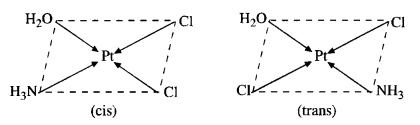Question 4.
Give evidence to show that [Co(NH3)5Cl]SO4 and [CO(NH3)5SO4]Cl exist as ionisation isomers.
Dissolve both the complexes separately in water. First add a few drops of BaCl2 solution to both these complexes. Only one of these will give white precipitate with the solution indicating that SO4 is not a part of complex entity. If exists as anion.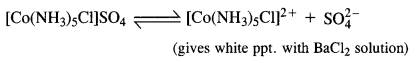Now again add a few drops of AgN03 solution to both these complexes. Only one of these will give white precipitate with the solution indicating that in this case Cl is not a part of complex entity. It exists as anion.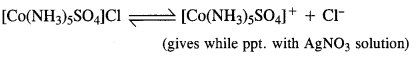This shows that the complexes exist as pair of ionisation isomers.

Question 5.
Explain on the basis of valence bond theory that [Ni(CN)4]2- ion with square planar structure is diamagnetic and the[Ni(CN)4]2- ion with tetrahedral geometry is paramagnetic.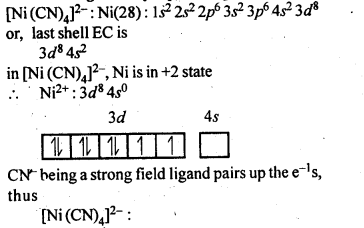Question 6.
[MCl4]2- is paramagnetic while [Ni(CO)4] is diamagnetic though both are tetrahedral. Why ? (C.B.S.E. Outside Delhi2012)
In the complex [NiCl4]2-, Ni is in + 2 oxidation state and has the configuration 3d840. The CL ion being a weak ligand cannot pair the two unpaired electrons present in 3d orbitals. This means that 3d orbitals are not involved in hybridisation. The complex is sp3 hybridised (tetrahedral) and is paramagnetic in nature. In the other complex [Ni(CO)4], the oxidation state of Ni is zero and electronic configuration is 3d84s2. In the presence of the ligand CO, the 4s electrons shift to the two half filled 3d orbitals and make all the electrons paired. The valence 4s and 3p orbitals are involved in hybridisation. The complex is tetrahedral but diamagnetic. For more details, consult text part.

Question 7.
[Fe(H2O)6]3+ is strongly paramagnetic whereas [Fe(CN)6]3- is weakly paramagnetic. Explain.
Outer electronic configuration of iron (Z = 26) in ground state is 3d64s2. Iron in this complex is in +3 oxidation state. Iron achieves +3 oxidation state by the loss of two 4s electrons and one 3d electron. The resulting Fe3+ ion has outer electronic configuration of 3d5. Since H2O is not a strong field ligand, it is unable to cause the electron pairing.
Outer electronic configuration of iron (Z=26) in ground state is 3d64s2. Iron in this complex is in +3 oxidation state. Iron achieves +3 oxidation state by the loss of two 4s electrons and one 3d electron. The resulting Fe3+ ion has outer electronic configuration as 3d5. CN ion is a strong field ligand

Question 8.
Explain [Co(NH3)6]3+ is an inner orbital complex while [Ni(NH3)6]2+ is an outer orbital complex.
In the complex [Co(NH3)6]3+, the oxidation state of cobalt is +3 and has 3d6 configuration. In the presence of NH3 molecules (ligands), two 3d electrons pair up and two 3d orbitals remain empty. Since six ligands are to be accommodated the hybridisation of the metal ion is d2sp3.As inner d-electrons are involved, the complex is inner orbital complex and is diamagnetic in nature.
In the complex [Ni(NH3)6]3 + , the oxidation state of Ni is +2 and has 3d8 configuration. Since six NH3 molecules (ligands) are to be accomodated, the hybridisation of metal ion is sp3d2. This means that 4d orbitals are involved in the hybridisation.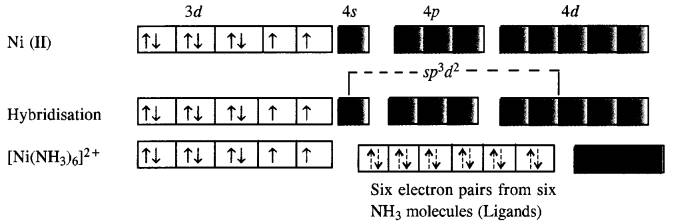The complex is paramagnetic as well as outer orbital complex since outer (4d) electrons are involved in the hybridisation.

Question 9.
Predict the number of unpaired electrons in the square planar [Pt(CN)4]2- ion.
The element Pt(Z = 78) is present in group 10 with electronic configuration 5d96s1. The divalent cation Pt2+ has 5d8 configuration.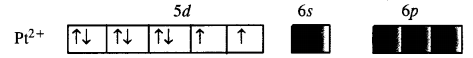For square planar complex, Pt (II) is in dsp2 hybridisation state. To achieve this, the two unpaired electrons present in 5d orbitals get paired. The complex has therefore, no unpaired electrons.

Question 10.
The hexaquomanganese(II) ion contains five unpaired electrons, while the hexacyano ion contains only one unpaired electron. Explain using Crystal Field Theory.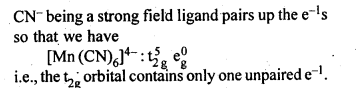Question 11.
Calculate the overall complex dissociation equilibrium constant for [Cu(NH3)4]2+ ion, given that p4 for the complex is 2·1 x 1013.
The dissociation constant is the reciprocal of overall stability constant (β4)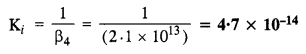NCERT EXERCISE

Question 1.
Explain bonding in co-ordination compounds in terms of Werner’s postulates.
The valencies of the elements in cobalt (III) chloride and in ammonia seem to be completely satisfied. However, the two substances react to form a stable compound with the formula COCl3·6NH3. The compound is known as co-ordination compound.

1. In co-ordination compounds, metal atoms exhibit two types of valencies. These are primary and secondary valencies.
2. The ligands attached to the primary valencies are ionisable and they represent the oxidation state of the metal. The ligands attached to the secondary valencies are non-ionisable and these correspond to its co-ordination number.
3. Every metal has fixed number of secondary valencies because its co-ordination number is fixed.
4. A metal tends to satisfy both these valencies. The primary valencies are satisfied by negative ions (anions) whereas secondary valencies are satisfied by either negative ions or neutral molecules.

Question 2.
FeSO4 solution mixed with (NH4)2SO4solution in 1:1 molar ratio gives the test of, Fe2+ion but CuSO4solution mixed with aqueous ammonia in 1:4 molar ratio does not give the test of Cu2+ ion. Explain why.
FeSO4 solution mixed with (NH4),SO4 solution in 1 : 1 molar ratio forms a double salt, FeS04 (NH4)2SO4-6H2O (Mohr’s salt) which ionizes in the solution to give Fe2+ions. Hence it gives the tests of Fe2+ ions. CuSO4 solution mixed with aqueous ammonia in 1:4 molar ratio forms a complex salt, with the formula [CU(NH3)4]SO4. The complex ion [Cu(NH3)4]2+ does not ionize to give Cu2+ ions. Hence, it does not give the tests of Cu2+ ion.

Question 3.
Explain with two examples each of the following : coordination entity, ligand, coordination number, coordination polyhedron, homoleptic and heterolepic.
(a) Co-ordination Entity: A coordination complex or entity is the species enclosed in square bracket. It is also called co-ordinate sphere. It contains in it a certain metal atom or ion to which a fixed number of neutral molecules or ions capable of donating electron pairs are linked with co-ordinate bonds. e.g. [COCl3(NH3)3]

(b) Ligand: Ligands are the electron donor molecules or ions which may be either neutral, or anionic (sometimes cationic as well) and are linked to the central metal atom or ion by co-ordinate bonds also called dative bonds. In fact, the electrons needed for the bond are provided by the ligands. e.g. H2NCH2CH2NH2 or N(CH2CH2NH2)3

(c) Co-ordination Number: The coordination number [C.N.] of a metal ion in a complex can be defined as the number of ligand or donor atoms to which the metal is directly bonded. For example, in the complex ions, [PtCl6]2- and [Ni(NH3)4]2+, the coordination number of Pt and Ni are 6 and 4 respectively. Similarly, in the complex ions, [Fe(C2O4)3]3- and [CO(en)3]3+,the coordination number of both, Fe and Co, is 6 because C2O42-and en (ethane-1,2-diamine) are bidentate ligands.

(d) Coordination polyhedron : The spatial arrangement of the ligand atoms which are directly attached to the central atom/ion defines a coordination polyhedron about the central atom. The most common coordination polyhedra are octahedral, square planar and tetrahedral. For example, [CO(NH3)6]3+ is octahedral, [Ni(CO)4] is tetrahedral and [PtCl4]2- is square planar.

(e) Homoleptic and heteroleptic complexe : Complexes in which a metal is bound to only one kind of donor groups, e.g., [CO(NH3)6]3+, are known as homoleptic. Complexes in which a metal is bound to more than one kind of donor groups, e.g., [CO(NH3)4Cl2]+, are known as heteroleptic.

Question 4.
What is meant by unidentate, didentate and ambidentate ligands ? Give two examples for each.
Unidentate ligands are those which bind to the metal ion through a single donor atom, e.g., Cl , H2O.

Bidentate ligands are those which bind to the metal ion through two donor atoms. e.g., ethane-1,2-diamine (H2NCH2CH2NH2), oxalate (C2O42-) ion.

Ambidentate ligands are those which can bind to metal ion through two different donor atoms, e.g., NO2 and SCN ion.

Question 5.
Specify the oxidation numbers of metals in the following co-ordination entities :
(a) [Co(H2O)(CN)(en2)]2+
(b) [PtCl4]2-
(c) [Cr(NH3)3Cl3]
(d) [CoBr2(en)2]+
(e) K3[Fe(CN)6].
(a) O.N. of Co : x + 0 + (-1) + 2(0)= + 2 or x = + 2+ 1 = + 3
(b) O.N. of Pt : x + 4 (-1) =-2 or x =-2 + 4 = + 2
(c) O.N. of Cr : x + 3(0) + 3(- 1) = 0 or x = + 3
(d) O.N. of Co : x + 2(- 1) + 2(0) = + 1 or x = + 1 + 2 = + 3
(e) O.N. of Fe : x + 6 (- 1) = – 3 or x = – 3 + 6 = + 3

Question 6.
Using IUPAC norms write the formulas for the following:
(i)Tetrahydroxozincate(Il)
(iii)Diamminedichlorido platinum (II)
(iv)Potassium tetracyanonickelate (II)
(v)Pentaamminenitrito-O-cobalt(III)
(vi)Ilexaamminccobalt (III) sulphate
(vii)Potassium tri(oxalato) chromate (III)
(yiii)Hexaammineplatinum (IV)
(ix)Tetrabromidocuprate(II)
(x) Pentaamminenitrito-N-cobalt (III)
(i)[Zn(OH)4]2- (ii)K2[PdCl4]
(iii)[Pt(NH3)2Cl2]
(iv)K2[Ni(CN)4]
(v)[Co(NH3)5(ONO)]2+
(vi)[Co(NH3)6]2(SO4)3
(vii)K3[Cr(C2O4)3]
(viii)[Pt(NH3)6]4+
(ix)[CuBr4]2-
(x)[Co(NH3)5(N02)]2+

Question 7.
Using IUPAC norms write the systematic names of the following :
(a) [CO(NH3)6]Cl3
(b) [CO(NH3)4Cl(NO2)]Cl
(c) [Ni(NH3)6]Cl2
(d) [Pt(NH3)2Cl(NH2CH3)]Cl
(e) [Mn(H2O)6]2+
(f) [NiCl4]2-
(g) [Co(en)3]3+
(h) [Ti(H2O)6]3+
(i) [Ni(CO)4]. (Jharkhand Board 2015)
(a) hexamminecobalt(III) chloride
(b) tetramminechloriodonitrito-N-cobalt(III) chloride
(c) hexaamminenickel(II) chloride
(d) diamminechlorido (methaneamine) platinum(II) chloride
(e) hexaaquamanganese(II) ion
(f) tetrachloriodonickelate(II) ion
(g) tris(ethane-l, 2-diammine) cobalt(III) ion
(h) hexaaquatitanium(III) ion
(i) tetracarbonylnickel (0).

Question 8.
List various types of isomerism possible for coordination compounds, giving an example of each.
(i) Geometrical isomerism: The isomer in which similar ligands occupy adjacent positions is referred to as cis isomer and the isomer in which similar ligands occupy opposite positions is referred to as trans isomer. Therefore, this type of isomerism is also known as cis-trans isomerism.(ii) Optical isomerism: The isomer which rotates the plane of polarised light to the right is called dextro rotatory and designated as d- and the one which rotates the plane of polarised light to the left is called laevo rotatory and designated as l. These optical isomers have identical physical and chemical properties except their behaviour towards the plane polarised light.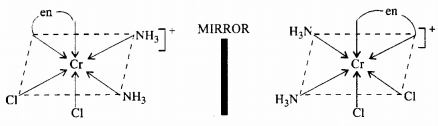(iii) Linkage isomerism: Linkage isomerism arises in a coordination compound containing ambidentate ligand. A simple example is provided by complexes containing the thiocyanate ligand, NCS, which may bind through the nitrogen to give M-N CS or through sulphur to give M-SCN. This behaviour was seen in the complex [CO(NH3)5(NO2)]Cl2, which is obtained as the red form, in which the nitrite ligand is bound through oxygen ( ONO), and as the yellow form, in which the nitrite ligand is bound through nitrogen (-NO2).

(iv) Coordination isomerism : This type of isomerism arises from the interchange of ligands between cationic and anionic entities of different metal ions present in a complex. An example is provided by [Co(NH3)6] [Cr(CN)6], in which the NH3 ligands are bound to CO3+ and the CN ligands to Cr3+. In its coordination isomer [Cr(NH3)6][CO(CN)6], the NH3 ligands are bound to Cr3+ and the CN ligands to CO3+.

(v) Ionisation isomerism : This form of isomerism arises when the counter ion in a complex salt is itself a potential ligand and can displace a ligand which can then become the counter ion. An example is provided by the ionisation isomers [CO(NH3)5SO4]Br and [CO(NH3)5Br]SO4.

(vi) Solvate isomerism : This form of isomerism is known as ‘hydrate isomerism in case where water is involved as a solvent. This is similar to ionisation isomerism. Solvate isomers differ by whether or not a solvent molecule is directly bonded to the metal ion or merely present as free solvent molecules in the crystal lattice. An example is provided by the aqua complex [Cr(H2O)6]Cl3 (violet) and its solvate isomer [Cr(H2O)5Cl]Cl2 H2O (grey green).

Question 9.
How many geometrical isomers are possible in the following coordination entities ?
(a) [Cr(C2O4)3]3-
(b) [Co(NH3)3Cl3]
(a) [Cr(C2O4)2]3- : No geometrical isomerism is possible.
(b) [CO(NH3)3Cl3] : Two geometrical isomers : fac and mer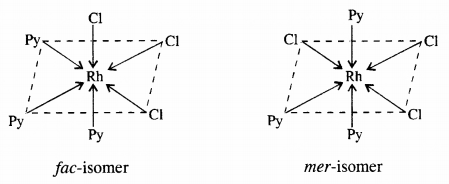Question 10.
Draw the structures of optical isomers of :
(a) [Cr(C2O4)3]3-
(b) [PtCl2(en)2]2+
(c) [Cr(NH3)2Cl2(en)]+ (C.B.S.E. Foreign 2015)Question 11.
Draw all the isomers (geometrical and optical) of :
(a) [CoCl2(en)2]+
(b) [CoNH3Cl(en)2]2+
(c) [Co(NH3)2Cl2(en)]+
(a)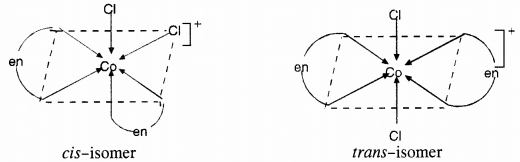(b)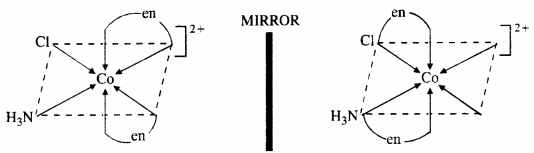(c)Question 12.
Write all the geometrical isomers of [Pt(NH3)(Br)(Cl)(py)]. How many of these will exhibit optical isomerism ?
There are three geometrical isomers.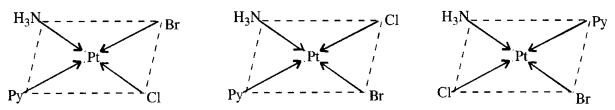Optical isomerism is generally not shown by the square planar complexes with CN = 4.

Question 13.
Aqueous copper sulphate solution (blue in colour) gives (a) green precipitate with aqueous potassium fluoride and (b) a bright green solution with aqueous potassium chloride solutions. Explain these experimental results.
Aqueous solution of copper sulphate which is blue in colour exists as [Cu(H2O)4]SO4 and gives [Cu(H2O)4]2+ in solution. It is a labile complex entity in which the ligands H2O get easily replaced by F ions of KF and by Cl ions of KCl.Question 14.
What is the coordination entity formed when excess of aqueous KCN is added to an aqueous solution of copper sulphate? Why is that no precipitate of copper sulphide is obtained when H2S(g) is passed through the solution?
On mixing the aqueous solutions of KCN and CuSO4, the complex formed is potassium tetracyanocuprate(II). Since CN” ions are strong ligands, the complex is quite stable. It is evident from the stability constant value (K = 2·0 x 1027). It is not cleaved by H2S gas when passed through the aqueous solution and no precipitate of CuS is formed.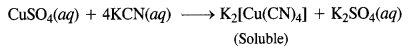Question 15.
Discuss the nature of bonding in the following co-ordination complexes on the basis of valence bond theory :
(a) [Fe(CN)6]4-
(b) [FeF6]3-
(c) [Co(C2O4)3]3-
(d) [CoF6]3-
(a) Hexacyanoferrate(II) ion [Fe(CN)6]4-: Iron in this complex is in + 2 oxidation state. Iron achieves + 2 oxidation state by the loss of two 4s electrons. The resulting Fe2+ ion has outer electronic configuration of 3d6.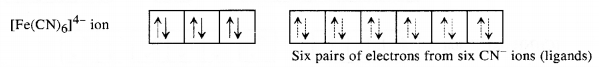(b) Hexafluoriodoferrate(II) ion [FeF6]3-: Iron in this complex is in +2 oxidation state. Iron achieves + 2 oxidation state by the loss of two 4s electrons. The resulting Fe2+ ion has outer electronic configuration of 3d6.(c)

(d) Hexafluorocobaltate(III) [CoF6]3-: Cobalt ion in the complex is in + 3 oxidation state. Cobalt achieves + 3 oxidation state by the loss of two 4s electrons and one 3d electron. The resulting Co3 + ion has outer electronic configuration of 3d6.Question 16.
Draw figure to show the splitting of d-orbitals in octahedral crystal field.
Let us assume that the six ligands are positioned symmetrically along the Cartesian axes, with metal atom at the origin.As the ligands approach, first there is an increase in energy of d-orbitals relative to that of the free ion just as would be the case in a spherical field.

The orbitals lying along the axes (dz2 and dx2 – y2) get repelled more strongly than dxy, dyz and dzx orbitals which have lobes directed between the axes.

The dz2 and dx2-y2 orbitals get raised in energy and dxy dyz, dxz orbitals are lowered in energy relative to the average energy in the spherical crystal field. Thus, the degenerate set of d-orbitals get split into two sets : the lower energy orbitals set, t2g and the higher energy orbitals set, eg. The energy is separated by ∆0

Question 17.
What is spectrochemical series? Explain the difference between a weak field ligand and a strong field ligand.
The arrangement of ligands in order of their increasing field strengths, i.e., increasing crystal field splitting energy (CFSE) values is called spertrochemical series.
The ligands with small value of CFSE (Δ0) are called weak field ligands whereas those with large value of CFSE (Δ0) are called strong field ligands.

Question 18.
What is crystal field splitting energy ? How does the magnitude of ∆0 decide the actual configuration of d- orbitals in a coordination entity ?
When ligands approach a particular metal ion, the d-orbitals split into two sets, one with lower energy while the other with higher energy. The difference of energy between the two sets orbitals is known as crystal field stabilising energy (CFSE).
(i) If ∆0 < P, the configuration will be t32ge1g and it is there in case of weak field ligands and high spin complex will be formed.
(ii) If ∆0 > P, the configuration will be t42ge0g and it is there in case of strong field ligands and low spin complex will be formed.

Question 19.
[Cr(NH3)6]3+ is paramagnetic while [Ni(CN)4]2- is diamagnetic. Explain why?
(i) Hexaamminechromium(III) ion [Cr(NH3)6]3+: Outer electronic configuration of chromium (Z=24) in ground state is 3d24s1 and in this complex, it is in the +3 oxidation state. Chromium achieves +3 oxidation state by the loss of one 4s electron and two 3d-electrons. The resulting Cr3+ ion has outer electronic configuration of 3d3.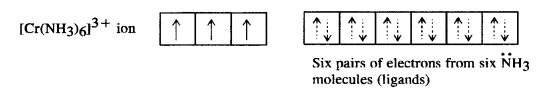(ii) Tetracyanonickelate (II) ion [Ni(CN)4]2-: Outer electronic configuration of nickel (Z = 28) in ground state is 3d84s2. Nickel in this complex is in + 2 oxidation state. It achieves + 2 oxidation state by the loss of the two 4s-electrons. The resulting Ni2+ ion has outer electronic configuration of 3d8.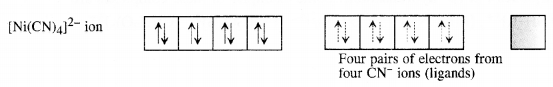Question 20.
A solution of [Ni(H2O)6]2+ is green but a solution of [Ni(CN)4]2- is colourless. Explain. (C.B.S.E. Delhi 2017)
Formation of [Ni(H2O)6]2+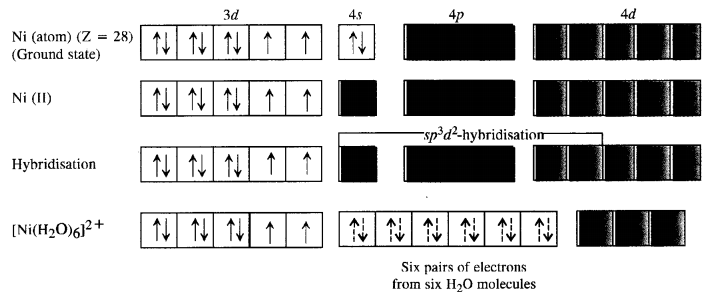Since H2P molecules represent weak field ligands, they donot cause any electron pairing. As a result the complex has two unpaired electrons and is coloured. The d-d transitions absorb radiations corresponding to red light and the complementary colour emitted is green.
Formation of [Ni(CN)4]2-. For the details of the structure of the complex,Since the complex has no unpaired electrons there is no scope for any d-d transition. The complex is therefore, colourless.

Question 21.
[Fe(CN)6]4- and [Fe(H2O)6]2+ are of different cdlours in dilute solutions. Why?
In both the complexes, Fe is in +2 oxidation state with cf configuration. This means that it has four unpaired electrons: Both CN ion and H20 molecules act as ligands occupy different relative positions in the spectrochemical series. They differ in crystal field splitting energy (Δ0). Quite obviously, they absorb radiations corresponding to different wavelengths and frequencies from the -visible region of light,
(VIBGYOR) and the transmitted colours are also different. This means that the complexes have different colours in solutions.

Question 22.
Discuss the nature of bonding in metal carbonyls.
The metal-carbon bond in metal carbonyls possess both s and p character. The M-C σ bond is formed by the donation of lone pair of electrons on the carbonyl carbon into a vacant orbital of the metal. The M-C π bond is formed by the donation of a pair of electrons from a filled d-orbital of metal into the vacant antibonding π* orbital of carbon monoxide. The metal to ligand bonding creates a synergic effect which strengthens the bond between CO and the metal.Question 23.
Give the oxidation state, d-orbital occupation and coordination number of the central metal ion in the following complexes :
(a) K3[CO(C2O4)3]
(b) (NH4)2[CoF4]
(C) Cis – [CrCl22(en)2]Cl
(d) [Mn(H2O)6]SO4
(a) OS = + 3, CN = 6, d-orbital occupation is 3d6 ({ t }_{ 2g }^{ 6 }{ e }_{ g }^{ 0 }),
(b) OS = + 2, CN = 4, 3d7 (({ t }_{ 2g }^{ 5 }{ e }_{ g }^{ 2 })),
(c) OS = + 3, CN = 6, 3d3 (({ t }_{ 2g }^{ 3 })),
(d) OS = + 2, CN = 6, 3d6 (({ t }_{ 2g }^{ 3 }{ e }_{ g }^{ 2 })).

Question 24.
Write down the IUPAC name for each of the following complexes and indicate the oxidation state, electronic configuration and coordination number. Also give stereochemistry and magnetic moment of the complex :
(a) K[Cr(H2O)2(C2O4)2]3H2O
(b) [CrCl3(py)3]
(c) K4[Mn(CN)6]
(d) [Co(NH3)5Cl]Cl2
(e) Cs[FeCl4]
(a) IUPAC name : potassium diaquadioxalatochromate (III) hydrate.
O.S. of Cr = + 3 ; 3d3 (({ t }_{ 2g }^{ 3 }{ e }_{ g }^{ 0 })) CN = 6 ; shape = octahedral, three unpaired electrons.
Magnetic moment (μ) = (sqrt { nleft( n+2 right) } =sqrt { 3times 5 } =sqrt { 15 } =3cdot 87BM)
(b) IUPAC name: trichloridotripyridinechromium (III) O.S. of Cr = + 3; 3 d3 (({ t }_{ 2g }^{ 3 }{ e }_{ g }^{ 0 })) CN = 6
shape = octahedral ; three unpaired electrons.
Magnetic moment (μ) = (sqrt { nleft( n+2 right) } =sqrt { 3times 5 } =3cdot 87BM)
(c) IUPAC name : potassiumhexacyanomanganate (II) O.S. of Mn = + 2 ; 3d5 (({ t }_{ 2g }^{ 5 }{ e }_{ g }^{ 0 })), CN = 6, shape = octahedral; one unpaired electron.
Magnetic moment (μ) = (sqrt { nleft( n+2 right) } =sqrt { 1times 3 } =sqrt { 3 } =1cdot 73BM)
(d) IUPAC name : pentaamminechloridocobalt (III) chloride
O.S. of Co = + 3 ; 3d6 (({ t }_{ 2g }^{ 6 }{ e }_{ g }^{ 0 })), CN = 6
shape = octahedral; zero unpaired electron. Magnetic moment (μ) = 0
(e) IUPAC name : cesium tetrachloridoferrate (III)
O.S. of Fe = + 3 ; 3d5 (({ e }^{ 2 }{ t }_{ 2 }^{ 3 })), CN = 4.
shape = tetrahedral ; five unpaired electrons.
Magnetic moment (μ) = (sqrt { nleft( n+2 right) } =sqrt { 5times 7 } =sqrt { 35 } =5cdot 92BM)

Question 25.
What is meant by stability of a coordination compound in solution ? State the factors which govern stability of complexes.
The stability of a complex in solution refers to the degree of association between the two species involved in the state of equilibrium. The magnitude of the (stability or formation) equilibrium constant for the association, quantitatively expresses the stability. Thus, if we have a reaction of the type :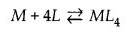then, larger the stability constant, the higher is the proportion of ML4 that exists in solution. Free metal ions rarely exist in the solution so that M will usually be surrounded by solvent molecules which will compete with the ligand molecules, L and be successively replaced by them. For simplicity, we generally ignore these solvent molecules and write four stability constants as follows :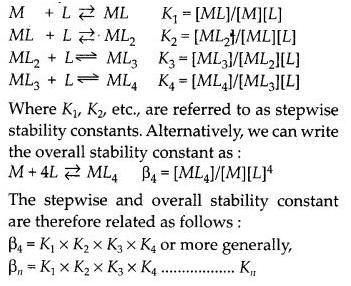Factors affecting stability of complexes :

1. Smaller the size of cation, greater will be the stability of complex e.g., Fe3+ forms more stable complex than Fe2+.
2. Greater the charge on central metal ion, more stable will be the complex e.g., Pt4+ forms more stable complex than Pt2+.
3. Stronger the ligand, more stable will be the complex formed e.g., CN forms more stable complex then NH3.

Question 26.
What is meant by chelate effect ? Give an example.
When a di-or polydentate ligand uses its two or more donor atoms to bind a single metal ion, it is said to be a chelate ligand. The number of such ligating groups is called the denticity of the ligand. Such complexes, called chelate complexes tend to be more stable than similar complexes containing unidentate ligands. Example : EDTA, DMG, etc.

Question 27.
Discuss briefly giving an example in each case the role of coordination compounds in:
(i) biological systems
(ii) medicinal chemistry
(iii) analytical chemistry and
(iv) extraction/ metallurgy of metals.
Application of coordination compounds.
(a) Used in qualitative and quantitative analysis.
(b) Determination of hardness of water
(c) Extraction of Au and Ag through complex formation
(d) Refining of Ni through complex formation
(e) Use of Rhodium complex in hydrogenation ofalkene.
(f) Use of EDTA in lead poisoning etc.

Question 28.
How many ions are produced from the complex Co(NH3)6Cl2 in solution?
(a) 6
(b) 4
(c) 3
(d) 2.
The complex will dissociate in aqueous solution to give three ions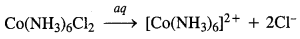Therefore, (c) is the correct answer.

Question 29.
Amongst the following ions which one has the highest magnetic movement value?
(a) [Cr(H2O)6]3+
(b) [Fe(H2O)6]2+
(c) [Zn(H2O)6]2+
The oxidation states of the metals in the complexes along with the electronic configuration are given :
(a) Cr3+ : 3d3 configuration ; unpaired electrons = 3
(b) Fe2+ : 3d6 configuration ; unpaired electrons = 4
(c) Zn2+ : 3d10 configuration ; unpaired electrons = 0
The complex (b) with maximum number of unpaired electrons has the highest magnetic moment. Therefore, (b) is the correct answer.

Question 30.
The oxidation number of cobalt in K[Co(CO)4] is
(a) + 1
(b) + 3
(c) – 1
(d) – 3.
O.N. of Co : x + 4(0) = -1 or x = -1. Therefore, (c) is the correct answer.

Question 31.
Amongst the following, the most stable complex is :
(a) [Fe(H2O)6]3+
(b) [Fe(NH3)6]3+
(c) [Fe(C2O4)3]3-
(d) [FeCl6]3-.
In all the complexes, Fe is in + 3 oxidation state. However, the complex (c) is a chelate because three ({ C }_{ 2 }{ O }_{ 4 }^{ 2- }) ions act as the chelating ligands. Thus, the most stable complex is (c).

Question 32.
What will be the correct order for the wavelengths of absorption in the visible region for the following : [Ni(NO2)6]4-,[Ni(NH3)6]2+,[Ni(H2O)6]2+.
In all the complexes, the metal ion is the same (Ni2+). The increasing field strengths of the ligands present as per electrochemical series are in the order :
H2O < NH3 < ({ NO }_{ 2 }^{ – })
The energies absorbed for excitation will be in the order :
[Ni(H2O)6]2+ < [Ni(NH3)6]2+ < [Ni(NO2)6]4-
As E = hc/λ i.e., E ∝ 1/λ; the wavelengths absorbed will be in the opposite order.

All Chapter NCERT Solutions For Class 12 Chemistry

—————————————————————————–

All Subject NCERT Solutions For Class 12

*************************************************

I think you got complete solutions for this chapter. If You have any queries regarding this chapter, please comment on the below section our subject teacher will answer you. We tried our best to give complete solutions so you got good marks in your exam.

If these solutions have helped you, you can also share ncertsolutionsfor.com to your friends.